## RaspEasy EXPERIMENTAL MODULE (by Didel)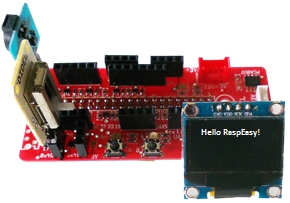Preliminary: This chapter can be used as independent introduction to sensor technology.
It contains some duplicated exercises from other chapters.

### Introduction

 In the previous chapters you learned how to wire and connect electronics parts to the Raspberry Pi, mostly using a breadboard. In principle the circuits were simple, but the Raspberry Pi can easily be damaged by small inattention (e.g. by applying more than 3.3V to a GPIO pin). Moreover you need to provide every electronic parts as single unit (buttons, LEDs, etc). To simplify basic experiments with the Raspberry Pi, we recommend to use a cheap, but well-thought-out add-on board by Didel, called RaspEasy. Simply insert the module into the 40-pin GPIO header and you are ready to go for funny and instructive programming adventures without the need to worry about connecting wires. Source of supply: http://www.didel.com Didel, CH-1092 Belmont, Switzerland Main features: 2 ADCs MCP3021 (10 bit, I2C) 2 pushbuttons 2 LEDs Grove/Minigrove connectors Overvoltage input protection Compatible extensions available (see Didel website for more information) (Seven-segment and Oled displays, potentiometer, active buzzer, etc.) Functional diagram:Header layout:### Experiment 1: Use a GPIO port as digital input or as digital output

 Aim: When the buttons A resp. B is pressed (hold down), the LED A resp. B lights up, when the button is released, the LED is dark. Program: [►] ```# RaspEasy1.py # Button press/release to switch on/off LED import RPi.GPIO as GPIO P_BUTTON = 12 # Button A #P_BUTTON = 13 # Button B P_LED = 7 # LED A #P_LED = 11 # LED B def setup(): GPIO.setmode(GPIO.BOARD) GPIO.setup(P_BUTTON, GPIO.IN) GPIO.setup(P_LED, GPIO.OUT) print "starting..." setup() while True: if GPIO.input(P_BUTTON) == GPIO.HIGH: GPIO.output(P_LED, GPIO.HIGH) else: GPIO.output(P_LED, GPIO.LOW) ``` Highlight program code(Ctrl+C copy, Ctrl+V paste) Remarks: There are two buttons A and B connected to pin #12 and #13 resp. When a button is released, the input pin is pulled down to ground by a resistor, when it is pressed, 3.3V is applied. Therefore there is no need for a software defined pull-up or pull-down resistor. The LED is turned on with a high output state (3.3V). This setup is sometimes called "positive-logic". In the example above, the state of the input pin is read ("scanned" or "polled") in a endless loop. The program is terminated by finishing the Python process. This is not a clean program termination because GPIO.cleanup() is never called. So some GPIO setups and states may survive until the next Python script invocation. Normally there is no harm in doing so. If you want to do better, have a look at experiment 4.

### Experiment 2: Button counter, contact bouncing

Aim:
A counter variable should be increased by one each time the button is pressed. The current count is written to the output area.

It's easy, isn't it? You simply adapt the program above and increase the counter when the button is pressed.

Program: [►]

```# RaspEasy2.py
# Button counter

import RPi.GPIO as GPIO

P_BUTTON = 12 # Button A

def setup():
GPIO.setmode(GPIO.BOARD)
GPIO.setup(P_BUTTON, GPIO.IN)

print "starting..."
setup()
count = 0
while True:
if GPIO.input(P_BUTTON) == GPIO.HIGH:
count += 1
print count
```
Highlight program code (Ctrl+C copy, Ctrl+V paste)

Remarks:
You see that you missed something. Each time you press the button, the count is increased many times not just 1. You get it quickly: The code runs through the count += 1 statement as long as the button is down. What is the remedy?

Best you consider the system as a state machine: There are two states: button-up and button-down. Only when the state changes from button-up to button-down the counter has to be increased. It is easy to handle the state by introducing a variable isButtonPressed that can be True or False (a so-called boolean variable). So your next attempt is the following:

Program: [►]

```# RaspEasy2a.py
# Button counter, 2nd attempt

import RPi.GPIO as GPIO

P_BUTTON = 12 # Button A

def setup():
GPIO.setmode(GPIO.BOARD)
GPIO.setup(P_BUTTON, GPIO.IN)

print "starting..."
setup()
count = 0
isButtonPressed = False
while True:
if GPIO.input(P_BUTTON) == GPIO.HIGH and not isButtonPressed:
isButtonPressed = True
count += 1
print count
elif GPIO.input(P_BUTTON) == GPIO.LOW and isButtonPressed:
isButtonPressed = False
```
Highlight program code (Ctrl+C copy, Ctrl+V paste)

Remarks:
This already works perfectly... but press rapidly with less force and you see that sometimes the count increases by two or three despite you pressed only once. This sporadic failure is very annoying and may spoil all your confidence in electronics and computing. What happens?

 When you close a mechanical switch, you make contact between two electrical conductors. If you do not apply enormous force when pressing the conductors together, the contact may reopen for a short while until it is definitely closed. So it is like closing the switch two (or several times). The same can happen when you release the switch: The contact may close again several times until it is definitely open. This effect, called switch bouncing, causes unpredictable results in a situation, where the number of closing or opening operations is important.You can experience switch bouncing in your experimental setup, escpecially while opening the contact. In your counting application is absolutely mandatory to eliminate switch bouncing. But how to do it?

You have two options: Either you add some extra electronics (actually a RS flip-flop or an RC-debouncer, search the Internet to inform you) or you do it by software. With software the idea to eliminate bouncing effects is the following:

 The repetition time of the GPIO.input() starting in the polling loop (so-called polling period) is quite small. When the software detects a high input signal for the first time, it considers it as the beginning of button press interval. The the polling is disabled about 100 ms and thus the extra low-high spikes are ignored.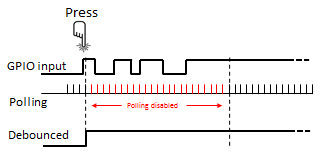After the button is released, when the first time the software considers the first low input detection as end of the press interval. Again the polling is disabled for about 100 ms and thus the extra low-high spikes are ignored.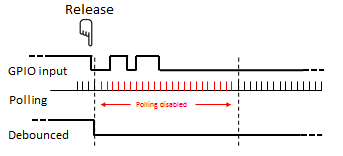Program: [►]

```# RaspEasy2b.py
# Button counter, 3rd attempt

import RPi.GPIO as GPIO
import time

P_BUTTON = 12 # Button A

def setup():
GPIO.setmode(GPIO.BOARD)
GPIO.setup(P_BUTTON, GPIO.IN)

print "starting..."
setup()
count = 0
isButtonPressed = False
while True:
if GPIO.input(P_BUTTON) == GPIO.HIGH and not isButtonPressed:
isButtonPressed = True
count += 1
print count
time.sleep(0.1)
elif GPIO.input(P_BUTTON) == GPIO.LOW and isButtonPressed:
isButtonPressed = False
time.sleep(0.1)
```
Highlight program code (Ctrl+C copy, Ctrl+V paste)

Remarks: As you see, we added the delay in the if and elif block. Is it not more elegant to put it once in the while block? This would work, but it is not completely equivalent, because a delay in the while block will be executed each time the loop is run, even in the "idle state" when the button is scanned to be pressed. So a short button hit that lasts less the 100 ms may be missed.

As you see, even the simple handling of a button requires a lot of computational thinking.

To get a standalone application you can display the counter value on an Oled or a 7-segment display.

Program: [►]

```# RaspEasy2c.py
# Button counter, use Oled or PyTell display

import RPi.GPIO as GPIO
import time
from OLED1306 import OLED1306
#from pytell import PyTell

P_BUTTON = 12 # Button A

def setup():
GPIO.setmode(GPIO.BOARD)
GPIO.setup(P_BUTTON, GPIO.IN)

print "starting..."
oled = OLED1306()
oled.setFontSize(50)
#pyTell = PyTell()

setup()
count = 0
oled.setText(str(count))
#  pyTell.showText("%4d" %count)
isButtonPressed = False
while True:
if GPIO.input(P_BUTTON) == GPIO.HIGH and not isButtonPressed:
isButtonPressed = True
count += 1
oled.setText(str(count))
#        pyTell.showText("%4d" %count)
time.sleep(0.1)
elif GPIO.input(P_BUTTON) == GPIO.LOW and isButtonPressed:
isButtonPressed = False
time.sleep(0.1)
```
Highlight program code (Ctrl+C copy, Ctrl+V paste)

Remarks: As you see, you must import either the OLED1306.py or the pytell.py module that you find in the code distribution.

### Experiment 3: LED state switcher

 Aim: When the button is pressed, the LED lights up and remains in the on-state until the button is pressed again; or in short: Click to turn the LED on and click to turn the LED off. Program: [►] ```# RaspEasy3.py # LED switcher import RPi.GPIO as GPIO import time P_BUTTON = 12 # Button A P_LED = 7 # LED A def setup(): GPIO.setmode(GPIO.BOARD) GPIO.setup(P_BUTTON, GPIO.IN) GPIO.setup(P_LED, GPIO.OUT) GPIO.output(P_LED, GPIO.LOW) # Turn off LED print "starting..." setup() isLedOn = False isButtonPressed = False while True: if GPIO.input(P_BUTTON) == GPIO.HIGH and not isButtonPressed: isButtonPressed = True if isLedOn: isLedOn = False GPIO.output(P_LED, GPIO.LOW) else: isLedOn = True GPIO.output(P_LED, GPIO.HIGH) time.sleep(0.1) elif GPIO.input(P_BUTTON) == GPIO.LOW and isButtonPressed: isButtonPressed = False time.sleep(0.1) ``` Highlight program code (Ctrl+C copy, Ctrl+V paste) Remarks: This experiment resembles to the button counter, but instead of a counter variable, the LED is used to indicate counter values 0 and 1 and instead of increasing the value of 1, it is reset to 0. Since the counter has only two values, it can be replaced by a boolean variable isLedOn = False or True. The variable is a "state variable" because it expresses the current state of the LED.

### Experiment 4: Start/Stop/Exit manager

 Aim: Buttons are often used as user input device to control the program. To make a demonstration, pressing the button A should start/stop a process, e.g. counting up. When button B is pressed, the program terminates. Program: [►] ```# RaspEasy4.py # Start/Stop, Terminate import RPi.GPIO as GPIO import time RUN_BUTTON = 12 # Button A EXIT_BUTTON = 13 # Button B def setup(): GPIO.setmode(GPIO.BOARD) GPIO.setup(RUN_BUTTON, GPIO.IN) GPIO.setup(EXIT_BUTTON, GPIO.IN) setup() count = 0 isCounting = False isRunButtonPressed = False print "Stopped" isExiting = False while not isExiting: if GPIO.input(RUN_BUTTON) == GPIO.HIGH and not isRunButtonPressed: isRunButtonRunPressed = True if not isCounting: isCounting = True print "Counting..." else: isCounting = False print "Stopped" time.sleep(0.1) elif GPIO.input(RUN_BUTTON) == GPIO.LOW and isRunButtonPressed: isRunButtonPressed = False time.sleep(0.1) if isCounting: count += 1 print count time.sleep(0.01) if GPIO.input(EXIT_BUTTON) == GPIO.HIGH: isExiting = True GPIO.cleanup() print "Programm terminated" ``` Highlight program code (Ctrl+C copy, Ctrl+V paste) Remarks: The program design is typical: A flag isExiting is used to test, if the program is executing the while-loop or should terminate by cleaning-up the GPIO handler.

### Experiment 5: Play Morse code with a buzzer

 Aim: An active buzzer performs like a LED. When the input signal is high, a sound of some predefined frequency and volume is emitted. Use an active buzzer to play some Morse code. Program: [►] ```# RaspiEx5.py import RPi.GPIO as GPIO import time #from OLED1306 import OLED1306 P_BUZZER = 40 # adapt to your wiring dt = 0.1 # adapt to your Morse speed morse = { 'a':'.-' , 'b':'-...' , 'c':'-.-.' , 'd':'-..' , 'e':'.' , 'f':'..-.' , 'g':'--.' , 'h':'....' , 'i':'..' , 'j':'.---' , 'k':'-.-' , 'l':'.-..' , 'm':'--' , 'n':'-.' , 'o':'---' , 'p':'.--.' , 'q':'--.-' , 'r':'.-.' , 's':'...' , 't':'-' , 'u':'..-' , 'v':'...-' , 'w':'.--' , 'x':'-..-' , 'y':'-.--' , 'z':'--..' , '1':'.----', '2':'..---', '3':'...--', '4':'....-', '5':'.....', '6':'-....', '7':'--...', '8':'---..', '9':'----.', '0':'-----', '-':'-....-', '?':'..--..', ',':'--..--', ':':'---...', '=':'-...-'} def s(n): # wait time.sleep(n * dt) def setup(): GPIO.setmode(GPIO.BOARD) GPIO.setup(P_BUZZER, GPIO.OUT) GPIO.output(P_BUZZER, GPIO.LOW) # Turn off buzzer def dot(): GPIO.output(P_BUZZER, GPIO.HIGH) s(1) GPIO.output(P_BUZZER, GPIO.LOW) s(1) def dash(): GPIO.output(P_BUZZER, GPIO.HIGH) s(3) GPIO.output(P_BUZZER, GPIO.LOW) s(1) def transmit(text): # sent = "" # initialize string buffer for c in text: # sent += c # oled.setText(sent) if c == " ": s(4) else: c = c.lower() if c in morse: k = morse[c] for x in k: if x == '.': dot() else: dash() s(2) print "starting..." #oled = OLED1306() #oled.setFontSize(16) setup() transmit("cq de hb9abh") GPIO.cleanup() print "all done" ``` Highlight program code (Ctrl+C copy, Ctrl+V paste) Remarks: A dictionary is used that defines the Morse code of each character as dot-dash sequence. The timing is very important. Consult the Internet to find out what are the timing requirements for standard Morse code. If you attach an Oled display, remove # from the out-commented lines of code. Then the the characters are shown the same time they are played. This gives you an additional interactivity, especially if you did not learn to decode Morse code by listening.

### Experiment 6: Using button events

 Programming with events may simplify the code considerably because it decouples several activities one from the other. The basic principle is the following: You define a so-called callback function (short: callback) that is never explicitly called by your program, but by the underlying system, whenever a predefined event happens. To enable the system to do this, you inform it with a so-called registration call by passing the function name. Event programming may also lead to unpredictable behavior, because the callback is mostly called in a separate thread that may conflict with other program threads. Aim: Write an event driven version of the button counter program. Each time the button is hit, the counter should increase by one. The Python GPIO module supports event programming. With add_event_detect() you register the callback and tell the system the port number and the transition type that triggers the event (LOW to HIGH, HIGH to LOW or both). Program: [►] ```# RaspEasy6.py # Using button events import RPi.GPIO as GPIO import time COUNT_BUTTON = 12 # Button A EXIT_BUTTON = 13 # Button B def onCountButtonEvent(channel): global count, btnReady if btnReady and GPIO.input(COUNT_BUTTON) == GPIO.HIGH: btnReady = False count += 1 else: btnReady = True time.sleep(0.1) def onExitButtonEvent(channel): global isExiting isExiting = True def setup(): GPIO.setmode(GPIO.BOARD) GPIO.setup(COUNT_BUTTON, GPIO.IN) GPIO.setup(EXIT_BUTTON, GPIO.IN) GPIO.add_event_detect(COUNT_BUTTON, GPIO.BOTH, onCountButtonEvent) GPIO.add_event_detect(EXIT_BUTTON, GPIO.BOTH, onExitButtonEvent) setup() btnReady = True count = 0 oldCount = 0 print "starting..." isExiting = False while not isExiting: if count != oldCount: oldCount = count print count time.sleep(0.001) GPIO.cleanup() print "Programm terminated" ``` Highlight program code (Ctrl+C copy, Ctrl+V paste) Remarks: We register both the button press and release events by using the GPIO.BOTH parameter value when registering the onCountButtonEvent callback using add_event_detect(). In contradiction to the documentation of the GPIO module, the additional parameter bouncetime in add_event_detect() does not completely eliminate switch bouncing. To avoid switch bouncing, we disable the press event by the btnReady flag until a button release event occurs. In addition we disable events for 0.1 s by calling time.sleep(0.1) in the callback, despite in gereral delays in callbacks should be omitted.

### Experiment 7: Reading analog data

Aim:
Most sensors output a voltage proportional (or in relation) to the physical value. To measure the value by a computer, the voltage has to be converted into an digital signal by a Analog-To-Digital converter (ADC). A potentiometer (variable resistor) can simulate the output of a sensor. It can be connected directly to the input ports of the RaspEasy ADC. Make a measurement every second and display the value using the print command.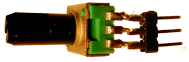Program: [►]

```# RaspEasy7.py

import smbus
import time

print "starting..."
bus = smbus.SMBus(1)

while True:
# Reads word (16 bits) as int
# Exchanges high and low bytes
data = ((rd & 0xFF) << 8) | ((rd & 0xFF00) >> 8)
# Ignores two least significiant bits
data = data >> 2
print "data:", data
time.sleep(1)
```
Highlight program code (Ctrl+C copy, Ctrl+V paste)

Remarks:
The MCP3021 is a ADC using the I2C protocol. Data are read and two bytes are transferred as an int using the read_word_data(address, cmd) function of the SMBus library (the cmd parameter is ignored and set to 0). Because the two bytes are in reverse order, they must be exchanged. As stated in the MC3021 datasheet, the result must then be shifted by two bits to the right to get rid of the two least significant bits.

This is low-level programming, but you may move the code into a function readData() and use it later without being concerned by the low-level details. This is typical for the procedural programming paradigm.

Program: [►]

```# RaspEasy7a.py

import smbus
import time

if port == 0:
elif port == 1:
data = ((rd & 0xFF) << 8) | ((rd & 0xFF00) >> 8)
data = data >> 2
return data

print "starting..."
bus = smbus.SMBus(1)
while True:
print "data:", v
time.sleep(1)
```
Highlight program code (Ctrl+C copy, Ctrl+V paste)

Remarks:
Using a sensor function readData() the program becomes extremely simple. You may show the sensor value on an attached display.

Program: [►]

```# RaspEasy7b.py

import smbus
import time
from OLED1306 import OLED1306
#from pytell import PyTell

if port == 0:
elif port == 1:
data = ((rd & 0xFF) << 8) | ((rd & 0xFF00) >> 8)
data = data >> 2
return data

print "starting..."
oled = OLED1306()
oled.setFontSize(50)
#pyTell = PyTell()

bus = smbus.SMBus(1)
while True:
oled.setText(str(v))
#    pyTell.showText("%4d" %v)
time.sleep(1)
```
Highlight program code (Ctrl+C copy, Ctrl+V paste)

Remarks:
Use the out-commented lines if you attach a PyTell 7-segment display. Now you know how to acquire analog data, you may use the RaspEasy board to read and display sensor values from many devices you have at hand.

### Experiment 8: Using a temperature sensor

Temperature measurement is very important in many situations, as consequence temperature sensors comes in many different flavors. In this exercise we use the LM35, a 3-pin integrated-circuit device with an output voltage that is in (almost) linear relation to the temperature and needs no calibration. Consult the datasheet for more information.

There is no need for additional components. Just connect the 3 connecting leads as shown here. ADC in is the input pin (center) of the RaspEasy ADC port A.The program displays the current temperature in degrees centigrade. Consider the following conversion: Since the sensor delivers a = 10 mV = 0.01 V per degrees centigrade, the output voltage is u = a * T. If the voltage u is applied to the 10-bit ADC powered with 3.3V, the digitized value returned to the program is v = u / 3.3 * 1023. So the relation between the temperature and the value returned from the ADC is v = 0.01 / 3.3 * 1023 * T = 3.1 * T.

Program: [►]

```# RaspEasy7c.py
# LM35 temperature sensor

import smbus
import time
#from OLED1306 import OLED1306

if port == 0:
elif port == 1:
data = ((rd & 0xFF) << 8) | ((rd & 0xFF00) >> 8)
data = data >> 2
return data

print "starting..."
#oled = OLED1306()
#oled.setFontSize(50)

bus = smbus.SMBus(1)
while True:
T = v / 3.1
print "T = %4.1f centigrades" %T
#    oled.setText(str(int(T + 0.5))) # rounded to int
time.sleep(1)
```
Highlight program code (Ctrl+C copy, Ctrl+V paste)

### Experiment 9: Using a light sensor (LDR)

A LDR (Light Dependent Resistor) is very simple to interface. Just solder the LDR with a 1kOhm resistor to a 3 pin male header and insert it into ADC port A or B.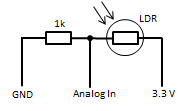You have only to modify a few lines in the program RaspEasy7c.py to make your first tests.

Program: [►]

```# RaspEasy7d.py
# LDR sensor

import smbus
import time
#from OLED1306 import OLED1306

if port == 0:
elif port == 1:
data = ((rd & 0xFF) << 8) | ((rd & 0xFF00) >> 8)
data = data >> 2
return data

print "starting..."
#oled = OLED1306()
#oled.setFontSize(50)

bus = smbus.SMBus(1)
while True: